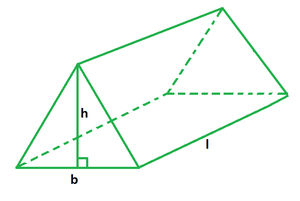Open in App
Not now

# Surface Area of a Triangular Prism Formula

• Last Updated : 26 Apr, 2022

A prism is a three-dimensional solid shape with two ends that are identical. It has flat sides, identical bases, and equal cross-sections. It has faces that are either parallelograms or rectangles with no bases. A triangular prism is one with three rectangular sides and two parallel triangle bases. The triangle bases are joined together by lateral sides that run parallel to one other.### Surface Area of a Triangular Prism Formula

A triangular prism’s surface area is also known as its total surface area. It is equal to the sum of the areas of all its faces. Knowing the base, length and height of a triangular prism is all that is required to calculate its surface area. The total surface area of a triangular prism is equal to the sum of twice the base area and thrice the product of a triangular base with the prism’s length.

Formula

A = bh + 3bl

where,

b is the triangular base,

h is the altitude of triangular base,

l is the prism’s length

### Sample Problems

Problem 1. Find the total surface area of a triangular prism if its base is 6 cm, altitude is 8 cm and length is 12 cm.

Solution:

We have, b = 6, h = 8 and l = 12.

Using the formula we have,

A = bh + 3bl

= 6 (8) + 3 (6) (12)

= 48 + 216

= 248 sq. cm

Problem 2. Find the total surface area of a triangular prism if its base is 5 cm, altitude is 7 cm and length is 8 cm.

Solution:

We have, b = 5, h = 7 and l = 8.

Using the formula we have,

A = bh + 3bl

= 5 (7) + 3 (5) (8)

= 35 + 120

= 155 sq. cm

Problem 3. Find the length of the triangular prism if its base is 6 cm, altitude is 9 cm and the area is 198 sq. cm.

Solution:

We have, b = 6, h = 9 and A = 198.

A = bh + 3bl

=> 198 = 6 (9) + 3 (6) (l)

=> 198 = 54 + 18l

=> 18l = 144

=> l = 8 cm

Problem 4. Find the altitude of the triangular prism if its base is 8 cm, length is 14 cm and volume is 408 sq. cm.

Solution:

We have, b = 8, l = 14 and A = 408

Using the formula we have,

A = bh + 3bl

=> 408 = 8h + 3 (8) (14)

=> 408 = 8h + 336

=> 8h = 72

=> h = 9 cm

Problem 5. Find the area of the base of the triangular prism if its length is 16 cm, height is 12 cm and the area is 480 sq. cm.

Solution:

We have, l = 16, h = 12 and A = 480.

Using the formula for volume we have,

A = bh + 3bl

A = b (h + 3l)

=> 480 = b (12 + 3 (16))

=> 60b = 480

=> b = 8 cm

Hence, the area of triangular base is,

A = (1/2) × b × h

= (1/2) × 8 × 12

= 48 sq. cm

My Personal Notes arrow_drop_up
Related Articles